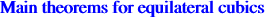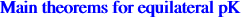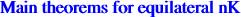A cubic is said to be equilateral when it has three real asymptotes making 60° angles with one another. When these asymptotes concur on the cubic, it is called a stelloid, a cubic whose Laplacian identically vanished. The most remarkable equilateral cubics are the McCay cubic and the Kjp cubic which are isogonal cubics. A study of these cubics can be found in Special Isocubics where many examples are provided. Here is a short summary of the results found in it :A general equilateral cubic is said to be a K60. When its asymptotes are concurrent, it is a K60+ (also called a stelloid) and when they concur on the curve, it is a K60++. Theorem 1 : a cubic (circumscribed or not) is a K60 if and only if the polar conics of two distinct infinite points are rectangular hyperbolas. Theorem 2 : a cubic K has three concurring asymptotes if and only if the polar lines of three distinct infinite points are themselves concurrent. In this case, the cubic is said to be a K+. Theorem 3 : a circum-cubic is a K60+ if and only if the polar conics of A, B, C are rectangular hyperbolas.Theorem 1 : for a given isoconjugation, there is one and only one pK60. Furthermore, this cubic is a pK60+ if and only if the pole of the isoconjugation lies on a certain cubic called Co in Special Isocubics (see §6.1). Theorem 2 : there are only two pK60++ : the Fermats cubics.Let Po = X(1989) be the isogonal conjugate of X(323). Theorem 1 : for a given isoconjugation, whose pole is not Po, all nK60 form a pencil of cubics all having the same asymptotic directions. Among them there is one and only one nK60+. In particular, there is only one isogonal nK60+ (the Kjp cubic) and only one isotomic nK60+. Theorem 2 : when the pole of the isoconjugation is Po, each point P of the plane is the root of a nK60. Furthermore, the cubic becomes a nK60+ if and only if P lies on the perpendicular bisector of OH.